## Convolution

A convolution is an integral which expresses the amount of overlap of one function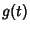as it is shifted over another function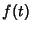. It therefore blends'' one function with another. For example, in synthesis imaging,the measured Dirty Map is a convolution of the true'' CLEAN Map with the Dirty Beam (the Fourier Transform of the sampling distribution). The convolution is sometimes also known by its German name, Faltung (folding''). A convolution over a finite range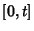is given by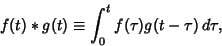(1)

where the symbol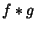(occasionally also written as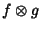) denotes convolution of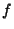and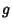. Convolution is more often taken over an infinite range,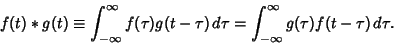(2)

Let,, and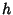be arbitrary functions anda constant. Convolution has the following properties: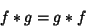(3)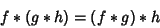(4)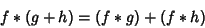(5)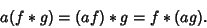(6)

The Integral identity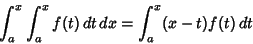(7)

also gives a convolution. Taking the Derivative of a convolution gives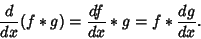(8)

The Area under a convolution is the product of areas under the factors,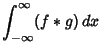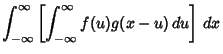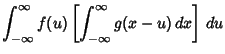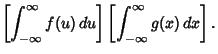(9)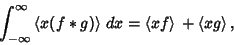(10)

as do the Variances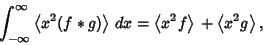(11)

where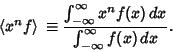(12)

ReferencesConvolution

Bracewell, R. Convolution.'' Ch. 3 in The Fourier Transform and Its Applications. New York: McGraw-Hill, pp. 25-50, 1965.

Hirschman, I. I. and Widder, D. V. The Convolution Transform. Princeton, NJ: Princeton University Press, 1955.

Morse, P. M. and Feshbach, H. Methods of Theoretical Physics, Part I. New York: McGraw-Hill, pp. 464-465, 1953.

Press, W. H.; Flannery, B. P.; Teukolsky, S. A.; and Vetterling, W. T. Convolution and Deconvolution Using the FFT.'' §13.1 in Numerical Recipes in FORTRAN: The Art of Scientific Computing, 2nd ed. Cambridge, England: Cambridge University Press, pp. 531-537, 1992.